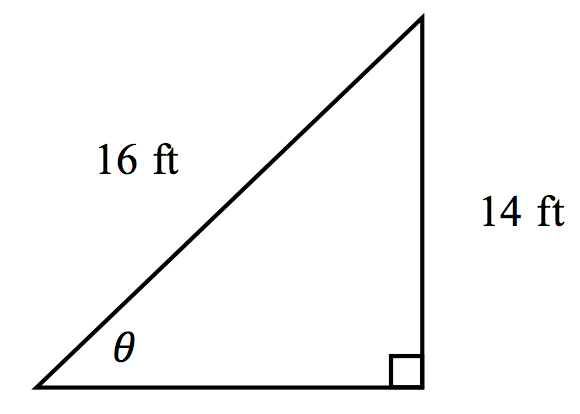### Home > INT2 > Chapter 5 > Lesson 5.1.4 > Problem5-46

5-46.

To paint a house, Travis leans a ladder against the wall. If the ladder is $16$ feet long and it makes contact with the house $14$ feet above ground, what angle does the ladder make with the ground? Draw a diagram of this situation and show all work.$\sin\theta=\frac{\text{opposite}}{\text{hypotenuse}}$

$\text{sin}\theta \ =\frac{14\text{ ft}}{16\text{ ft}}$

$\theta \ = \text{ sin}^{-1}\left(\frac{14\text{ ft}}{16\text{ ft}} \right )$

$θ\approx61^\circ$